Ex 11.1

Chapter 11 Class 9 Constructions [Deleted]
Serial order wise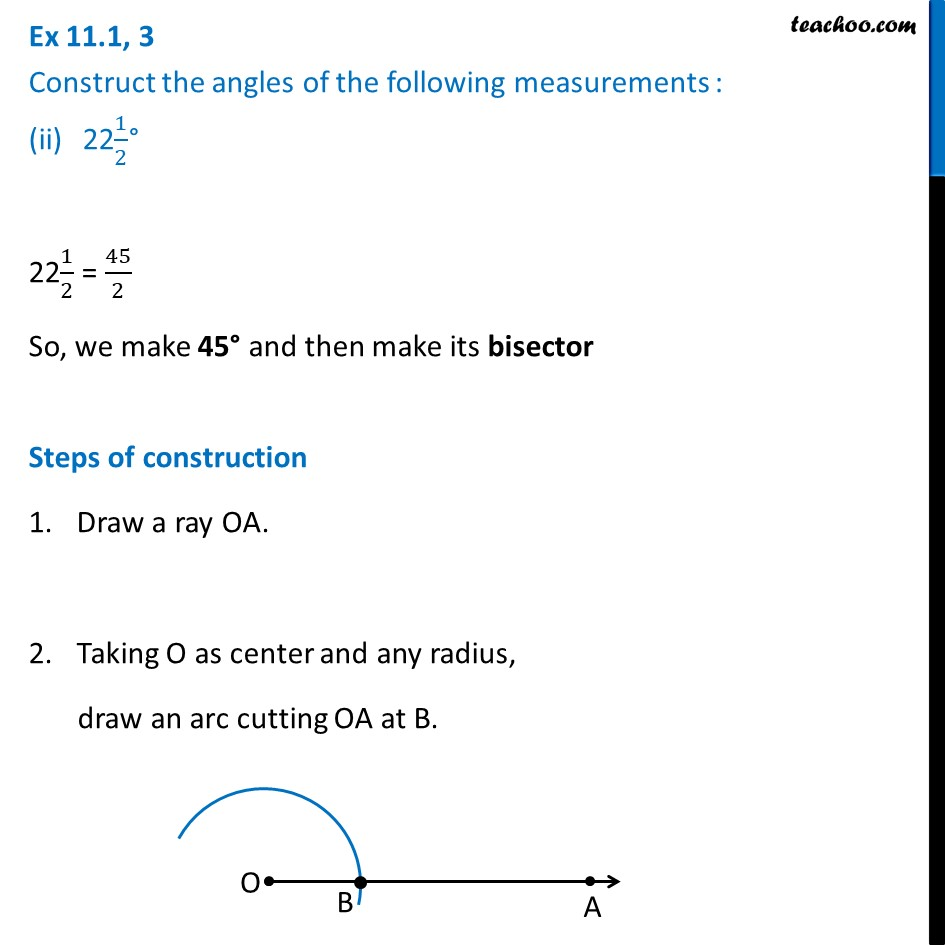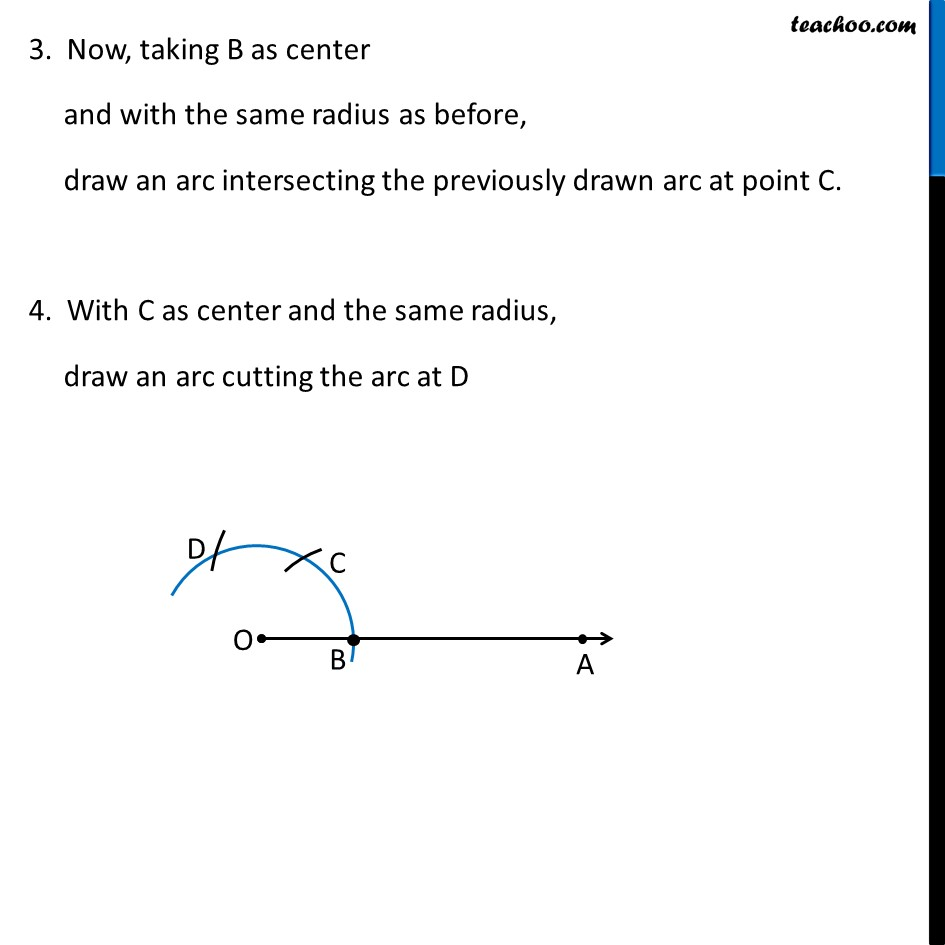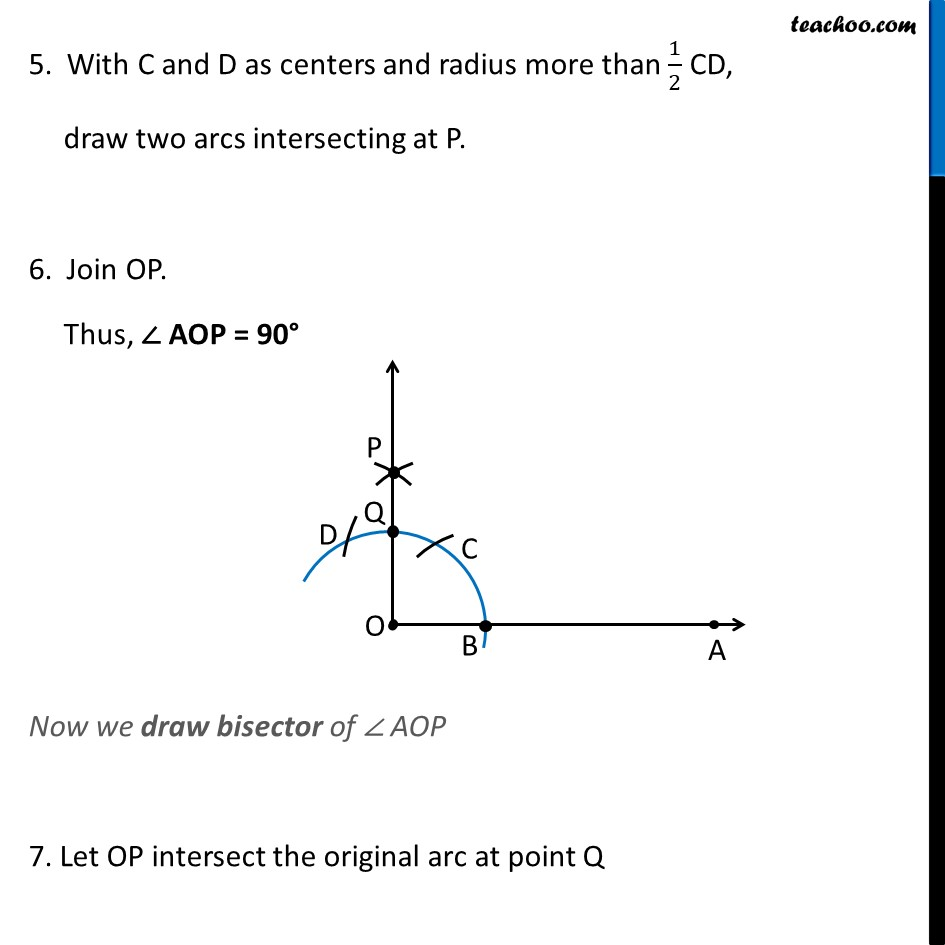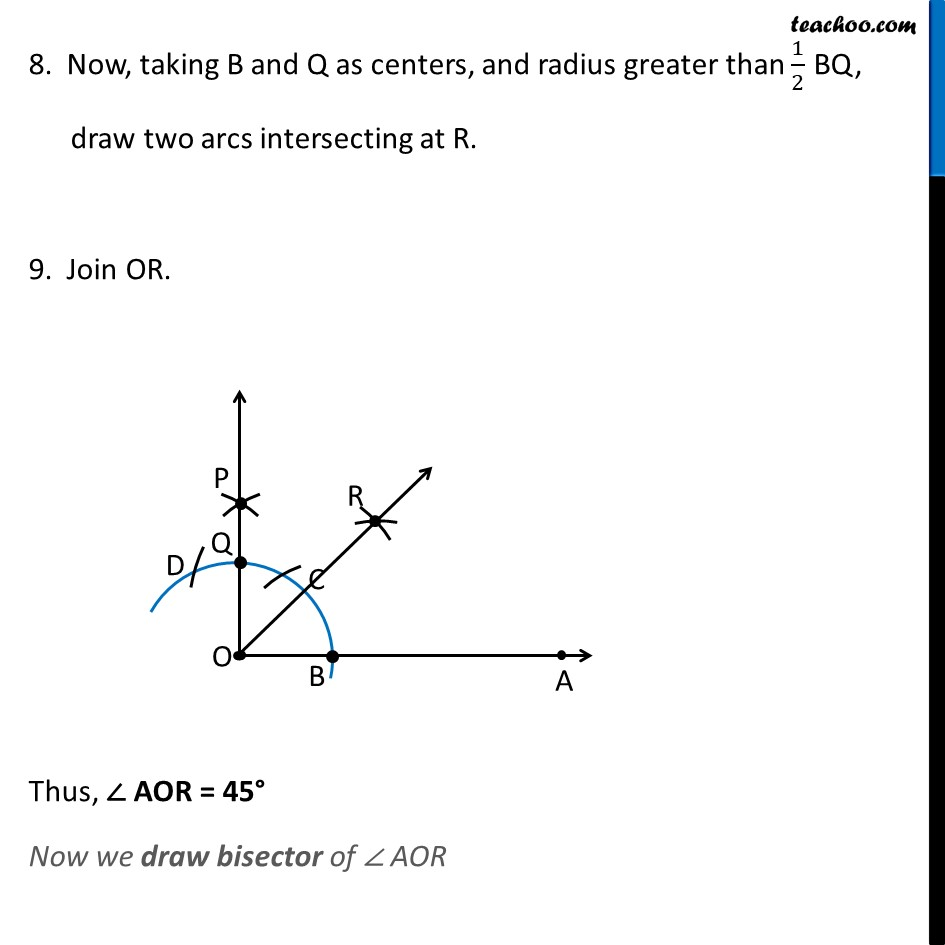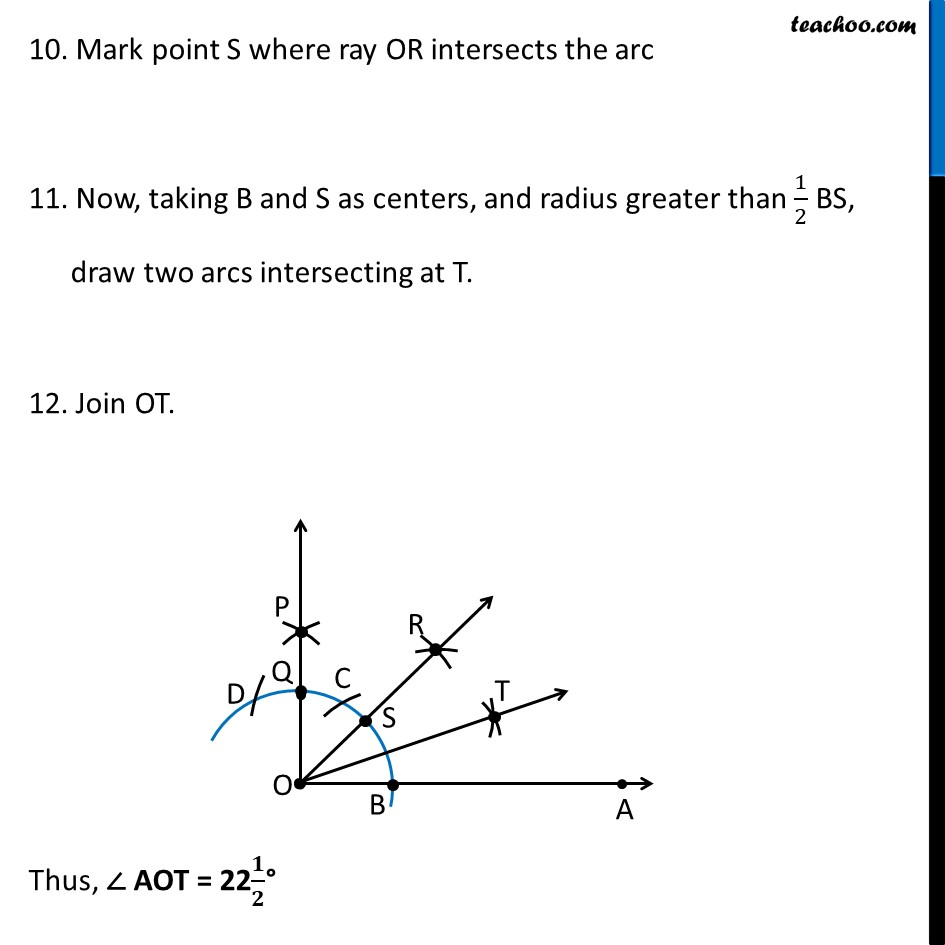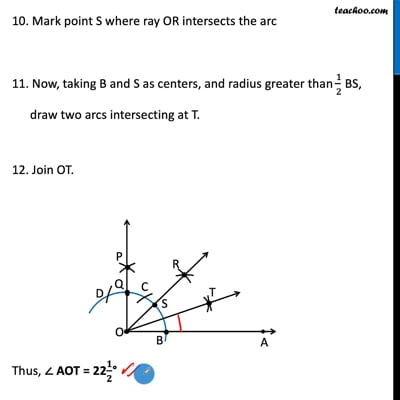This video is only available for Teachoo black users

Introducing your new favourite teacher - Teachoo Black, at only ₹83 per month

### Transcript

Ex 11.1, 3 Construct the angles of the following measurements : 221/2° 221/2 = 45/2 So, we make 45° and then make its bisector Steps of construction Draw a ray OA. Taking O as center and any radius, draw an arc cutting OA at B. 3. Now, taking B as center and with the same radius as before, draw an arc intersecting the previously drawn arc at point C. 4. With C as center and the same radius, draw an arc cutting the arc at D 5. With C and D as centers and radius more than 1/2 CD, draw two arcs intersecting at P. 6. Join OP. Thus, ∠ AOP = 90° Now we draw bisector of ∠ AOP 7. Let OP intersect the original arc at point Q 8. Now, taking B and Q as centers, and radius greater than 1/2 BQ, draw two arcs intersecting at R. 9. Join OR. Thus, ∠ AOR = 45° Now we draw bisector of ∠ AOR 10. Mark point S where ray OR intersects the arc 11. Now, taking B and S as centers, and radius greater than 1/2 BS, draw two arcs intersecting at T. 12. Join OT. Thus, ∠ AOT = 22𝟏/𝟐°